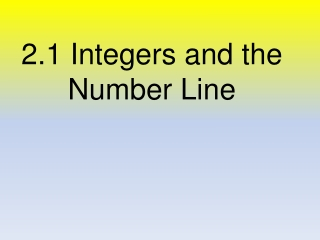DownloadDownload Presentation2.1 Integers and the Number Line

2.1 Integers and the Number Line

Télécharger la présentation2.1 Integers and the Number Line

- - - - - - - - - - - - - - - - - - - - - - - - - - - E N D - - - - - - - - - - - - - - - - - - - - - - - - - - -
Presentation Transcript

1. 2.1 Integers and the Number Line

2. What is an integer? • Can you name any integers?

3. Whole numbers: 0, 1, 2, 3, 4, … • Opposites: Numbers that are the same distance from zero, but on the opposite side of zero • Ex: 3 (“positive three”) and – 3 (“negative three”)

4. Integers: consists of the positive numbers, negative numbers, and zero {… - 4, -3, -2, -1, 0, 1, 2, 3, 4, …}

5. Name the integer that is suggested by each situation. • 1. Death valley is 282 ft below sea level • 2. The temperature is 3° below 0 • 3. The halfback made a gain of 8 yds • 4. Mikey's juice stand made an \$18 profit on Monday

6. Inequalities

7. Examples

8. Write a true sentence using > or < 2 ____ 9 -7 ____ 3 6 ____ -12 -18 ____ -15 -15 ____ -5 11 ____ -2

9. Absolute value • The distance from zero. • |n| = “the absolute value of n” • Turns negatives into positives • ALWAYS positive!!!

10. Examples 5. |3| 6. |-3| 7. |21| 8. |-14| • |12| • |-7| • |-3.04| • |0|

11. Answer the following questions using complete sentences. 1) How do you decide which number is greater when both numbers are positive? 2) How do you decide which number is greater when both numbers are negative? 3) How do you decide which number is greater when one number is positive and one number is negative?